# Solitons in Optical Fibers

Solitons form in optical fibers because a balance between the chirps induced by GVD and SPM, both of which limit the system performance when acting independently. To understand how such a balance is possible, we follow the analysis of the dispersion-induced pulse broadening tutorial and the nonlinear optical effects tutorial. As shown there, the GVD broadens an optical pulse during its propagation inside an optical fiber, except when the pulse is initially chirped in the right way. More specifically, a chirped pulse is compressed during earlier stages of propagation whenever β2 and the chirp parameter C happen to have opposite signs so that β2C is negative. As discussed in the nonlinear effects tutorial, SPM imposes a chirp on the optical pulse such that C > 0. If β2 < 0, the condition β2C < 0 is readily satisfied. Moreover, as the SPM-induced chirp is power-dependent, it is not difficult to imagine that under certain conditions, SPM and GVD may cooperate in such a way that the SPM-induced chirp is just right to cancel the GVD-induced broadening of the pulse. In this situation, an optical pulse propagates undistorted in the form of a soliton.

#### 1. Properties of Optical Solitons

To find the conditions under which solitons can form, we assume that pulses are propagating in the region of anomalous GVD and choose s = -1 in the equation below: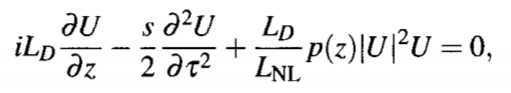where, depending on the sign of β2. We also set p(z) = 1, a condition requiring perfect distributed amplification. Introducing a normalized distance in the form ξ = z/LD, the equation above takes the form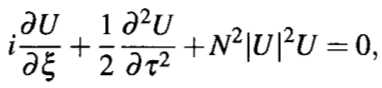where the parameter N is defined asIt represents a dimensionless combination of the pulse and fiber parameters. Even the single parameter N can be removed by introducing u = NU as a renormalized amplitude. With this change, the NLS equation takes on its canonical formThis NLS equation belongs to a special class of nonlinear partial differential equations that can be solved exactly with a mathematical technique known as the inverse scattering method. It was first solved in 1971 by this method. The main result can be summarized as follows. When an input pulse having an initial amplitude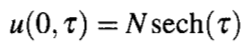is launched into the fiber, its shape remains unchanged during propagation when N = 1 but follows a periodic pattern for integer values of N > 1 such that the input shape is recovered at ξ = mπ/2, where m is an integer.

An optical pulse whose parameters satisfy the condition N = 1 is called the fundamental soliton. Pulses corresponding to other integer values of N are called higher-order solitons. The parameter N represents the order of the soliton. Noting that ξ = z/LD, the soliton period z0, defined as the distance over which higher-order solitons first recover their original shape, is given by z0 = (π/2)LD. The soliton period z0 and the soliton order N play an important role in the theory of optical solitons. The following figure shows the evolution of a third-order soliton over one soliton period by solving the NLS equation numerically with N = 3. The pulse shape changes considerably but returns to its original form at z = z0. Only a fundamental soliton maintains its shape during propagation inside optical fibers. In a 1973 study, the NLS equation was solved numerically to show that optical solitons can form in optical fibers.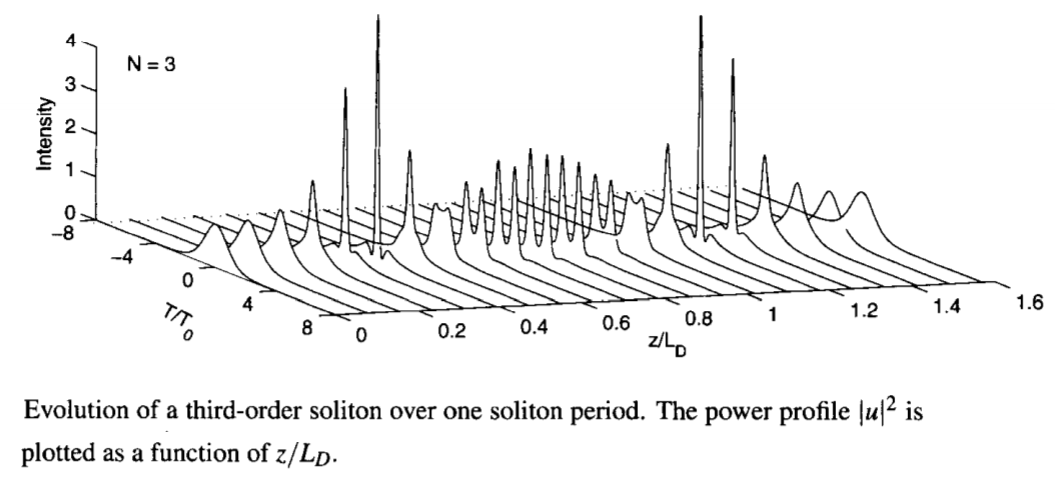The solution corresponding to the fundamental soliton can be obtained by solving the following equation directly, without recourse to the inverse scattering method.The approach consists of assuming that a solution of the form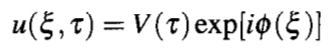exists, where V must be independent of ξ for this solution to represent a fundamental soliton that maintains its shape during propagation. The phase φ can depend on ξ but is assumed to be time-independent. When this solution is substituted in the NLS equation above and the real and imaginary parts are separated, we obtain two real equations for V and φ. These equations show that φ should be of the form φ(ξ) = Kξ, where K is a constant. The function V(τ) is then found to satisfy the nonlinear differential equation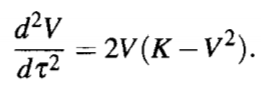This equation can be solved by multiplying it with 2(dV/dτ) and integrating over τ. The result is found to be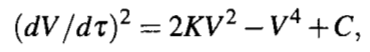where C is a constant of integration. Using the boundary condition that both V and dV/dτ should vanish for any optical pulse at |τ| -> ∞, C can be set to zero.

The constant K in the equation above is determined using the boundary condition that V = 1 and dV/dτ = 0 at the soliton peak, assumed to occur at τ = 0. Its use provides K = 1/2, resulting in φ = ξ/2. The equation above is easily integrated to obtain V(τ) = sech(τ). We have thus found the well-known "sech" solution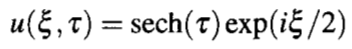for the fundamental soliton by integrating the NLS equation directly. It shows that the input pulse acquires a phase shift ξ/2 as it propagates inside the fiber, but its amplitude remains unchanged. It is this property of a fundamental soliton that makes it an ideal candidate for optical communications. In essence, the effects of fiber dispersion are exactly compensated by the fiber nonlinearity when the input pulse has a "sech" shape and its width and peak power are related by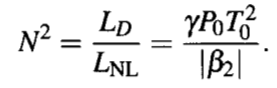in such a way that N = 1.

An important property of optical solitons is that they are remarkably stable against perturbations. Thus even though the fundamental soliton requires a specific shape and a certain peak power corresponding to N = 1 in the equation above, it can be created even when the pulse shape and the peak power deviate from the ideal conditions. The figure below shows the numerically simulated evolution of a Gaussian input pulse for which N = 1 but u(0, τ) = exp(- τ2/2). As seen there, the pulse adjusts its shape and width as it propagates down the fiber in an attempt to become a fundamental soliton and attains a "sech" profile for ξ ≫ 1. Similar behavior is observed when N deviates from 1. It turns out that the Nth-order soliton can form when the input value of N is in the range N - 1/2 to N + 1/2. In particular, the fundamental soliton can be excited for values of N in the range of 0.5 to 1.5.It is surprising that an optical fiber can force any input pulse to evolve toward a soliton. A simple way to understand this behavior is to think of optical solitons as the temporal modes of a nonlinear waveguide. Higher intensities int he pulse center create a temporal waveguide by increasing the refractive index only in the central part of the pulse. Such a waveguide supports temporal modes just as the core-cladding index difference leads to spatial modes of optical fibers. When the input pulse does not match a temporal mode precisely but is is close to it, most of the pulse energy can still be coupled to that temporal mode. The rest of the energy spreads in the form of dispersive waves. It will be seen later that such dispersive waves affect system performance and should be minimized by matching the input conditions as close to the ideal requirements as possible. When the solitons adapt to perturbations adiabatically, perturbation theory developed specifically for solitons can be used to study how the soliton amplitude, width, frequency, speed, and phase evolve along the fiber.

The NLS equation can be solved with the inverse scattering method even when an optical fiber exhibits normal dispersion. The intensity profile of the resulting solutions exhibits a dip in a uniform background, and it is the dip that remains unchanged during propagation inside an optical fiber. For this reason, such solutions of the NLS equation are called dark solitons. Even though dark solitons were observed during the 1980s and their properties have been studied thoroughly, most experiments have employed bright solitons with a "sech" shape. In the following discussion we focus on the fundamental soliton.

#### 2. Loss-Managed Solitons

We have seen in the preceding section that solitons use SPM to maintain their width even in the presence of fiber dispersion. However, this property holds only if soliton energy remains constant inside the fiber. It is not difficult to see that a decrease in pulse energy because of fiber losses would produce soliton broadening because a reduce peak power weakens the SPM effect necessary to counteract the GVD. When optical amplifiers are used periodically for compensating fiber losses, soliton energy changes in a periodic fashion. Such energy variations are included in the NLS equation through the periodic function p(z). In the case of lumped amplifiers, p(z) decreases exponentially between two amplifiers and can vary by 20 dB or more over each period. The important question is whether solitons can maintain their shape and width in spite of such large energy fluctuations. It turns out that solitons can remain stable over long distances, provided amplifier spacing LA is kept much smaller than the dispersion length LD.

In general, changes in soliton energy are accompanied by changes in pulse width. Large rapid variations in p(z) can destroy a soliton if its width changes rapidly through the emission of dispersive waves. The concept of the path-averaged soliton makes use of the fact that solitons evolve little over a distance that is short compared with the dispersion length (or soliton period). Thus, when LA ≪ LD, the width of a soliton remains virtually unchanged even if its peak power varies considerably in each section between two amplifiers. In effect, one can replace p(z) by its average value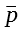when LA ≪ LD. Noting thatis just a constant that modifies γP0, we recover the standard NLS equation.

From a practical viewpoint, a fundamental soliton can be excited if the input peak power Ps (or energy) of the path-averaged soliton is chosen to be larger by a factor of. If we introduce the amplifier gain as G = exp(αLA) and usethe energy enhancement factor for loss-managed solitons is given byThus, soliton evolution in lossy fibers with periodic lumped amplification is identical to that in lossless fibers provided (1) amplifiers are spaced such that LA ≪ LD and (2) the launched peak power is larger by a factor fLM. As an example, G = 10 and fLM ≈ 2.56 when LA = 50 km and α = 0.2 dB/km.

The condition LA ≪ LD is vague for designing soliton systems. The question is how close LA can be to LD before the system may fail to work. The semi-analytic approach of last subsection can be extended to study how fiber losses affect the evolution of solitons. However, we should use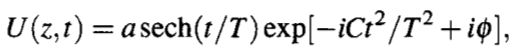to ensure that the "sech" shape of a soliton is maintained. Using the variational or the moment method, we obtain the following two coupled equations: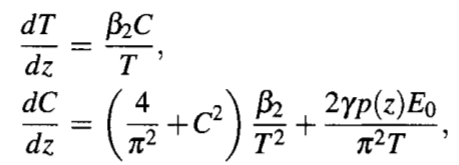where E0 = 2P0T0 is the input pulse energy. A comparison obtained for Gaussian pulses shows that the width equation remains unchanged; the chirp equation also has the same form but different coefficients.

As a simple application of the moment equations, we use them for finding the soliton-formation condition in the ideal case of p(z) = 1. If the pulse is initially unchirped, both derivatives in the two equations above vanish at z = 0 if β2 is negative and the pulse energy is chosen to be E0 = 2|β2|/(γT0). Under such conditions, the width and chirp of the pulse will not change with z, and the pulse will form a fundamental soliton. Using E0 = 2P0T0, it is easy to see that this condition is equivalent to setting N = 1.

To include fiber losses, we set p(z) = exp(- αz) in each fiber section of length LA in a periodic fashion. The figure below shows how the soliton width and chirp change at successive amplifiers for several values of LA in the range 25 to 100 km, assuming LD = 100 km. Such values of dispersion length are realized for a 10-Gb/s soliton system, for example, when T0 = 20 ps and β2 = -4 ps2/km. When amplifier spacing is 25 km, both the width and chirp remain close to their input values. As LA is increased to 50 km, they oscillate in a periodic fashion, and oscillation amplitude increases as LA increases. For example, the width can change more than 10% when LA = 75 km. The oscillatory behavior can be understood by performing a linear stability analysis. However, if LA/LD exceeds 1 considerably, the pulse width starts to increase exponentially in a monotonic fashion. The figure below shows that LA/LD ≤ 0.5 is a reasonable design criterion when lumped amplifiers are used for loss management.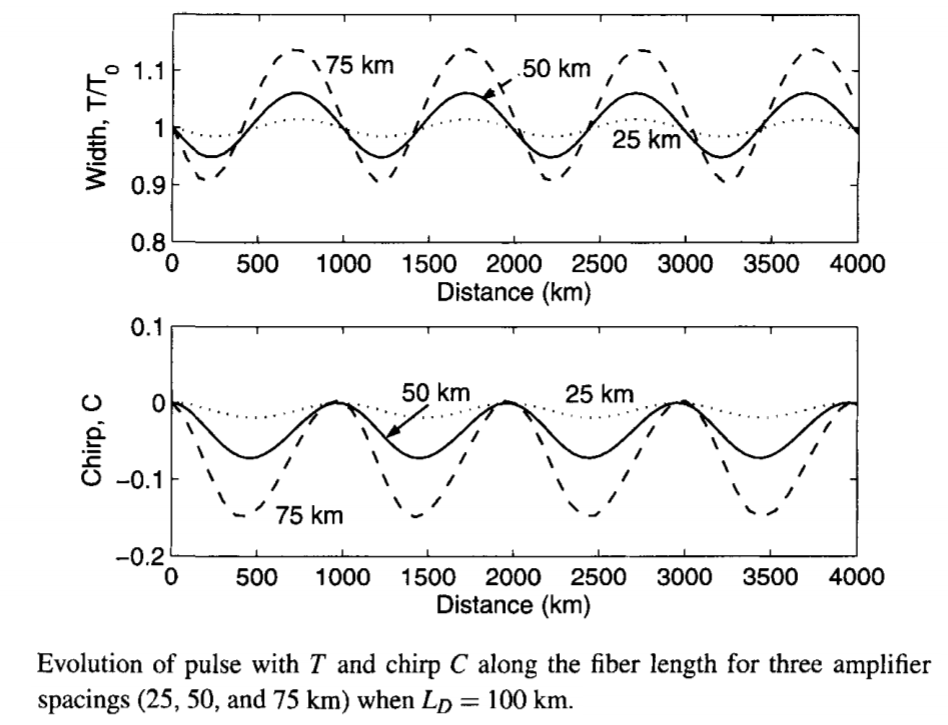The variational equations above only serve as a guideline, and their solutions are not always trustworthy, because they completely ignore the dispersive radiation generated as solitons are perturbed. For this reason, it is important to verify their predictions through direct numerical simulations of the NLS equation itself. The figure below shows the evolution of a loss-managed soliton over a distance of 10,000 km, assuming that solitons are amplified every 50 km. When the input pulse width corresponds to a dispersion length of 200 km, the soliton is preserved quite well even after 10,000 km because the condition LA ≪ LD is well satisfied. However, if the dispersion length is reduce to 25 km, the soliton is unable to sustain itself because of the excessive emission of dispersive waves.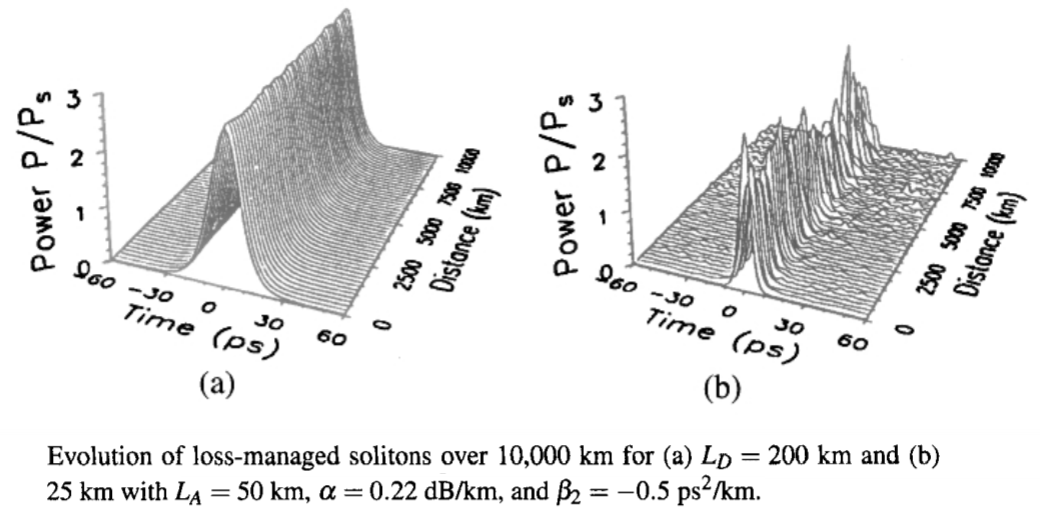How does the limitation on amplifier spacing affect the design of soliton systems? The condition LA ≪ LD can be related to the width T0 through LD = T02/|β2|. The resulting condition is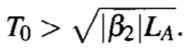The pulse width T0 must be a small fraction of the bit slot Tb = 1/B to ensure that the neighboring solitons are well separated. Mathematically, the soliton solution is valid only when a single pulse propagates by itself. It remains approximately valid for train of pulses only when individual solitons are well isolated. This requirement can be used to relate the soliton with T0 to the bit rate B using Tb = 2q0T0, where 2q0 is a measure of separation between two neighboring pulses in an optical bit stream. Typically, q0 exceeds 4 to ensure that pulse tails do not overlap significantly. Using T0 = (2q0B)-1, we obtain the following design criterion: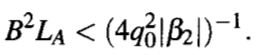Choosing typical values, β2 = -2 ps2/km, LA = 50 km, and q0 = 5, we obtain T0 > 10 ps and B < 10 GHz. Clearly, the use of path-averaged solitons imposes a severe limitation on both the bit rate and the amplifier spacing for soliton communication systems. To operate even at 10 Gb/s, one must reduce either q0 or LA if β2 is kept fixed. Both of these parameters cannot be reduced much below the values used in obtaining the preceding estimate.

A partial solution to this problem was suggested in 2000 when it was proposed that one could prechirp the soliton to relax the condition LA ≪ LD, even though the standard soliton solution has no chirp. The basic idea consists of finding a periodic solution that repeats itself at each amplifier using the periodic boundary conditions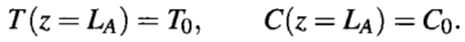The input pulse energy E0 and input chirp C0 can be used as two adjustable parameters. A perturbative solution shows that the pulse energy must be increased by a factor close to the energy enhancement factor fLM. At the same time, the input chirp that provides a periodic solution is related to this factor as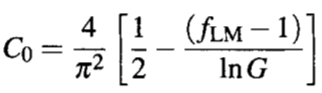Numerical results based on the NLS equation show that with a proper prechirping of input solitons, amplifier spacing can exceed 2LD. However, dispersive waves eventually destabilize a soliton over long fiber lengths when LA is made significantly larger than the dispersive length.

The condition LA ≪ LD can also be relaxed considerably by employing distributed amplification. A distributed-amplification scheme is superior to lumped amplification because its use provides a nearly lossless fiber by compensating losses locally at every point along the fiber link. As early as 1988, solitons were transmitted over 4,000 km of lossy fiber using periodic Raman amplification inside a 42-km recirculating fiber loop. The distance could later be increased to 6,000 km with further optimization. This experiment was the first to demonstrate that solitons could be transmitted over transoceanic distances in principle. Starting in 1989, lumped amplifiers were used for loss-managed soliton systems. In a 1991 experiment, 2.5-Gb/s solitons were transmitted over 12,000 km by using a 75-km recirculating loop. In this experiment, the performance was limited mainly by the timing jitter induced by amplifier noise. During the 1990s, several schemes for reducing the timing jitter were discovered and employed for improving the performance of soliton systems. The technique of Raman amplification has become quite common in recent years for both the soliton and nonsoliton systems.